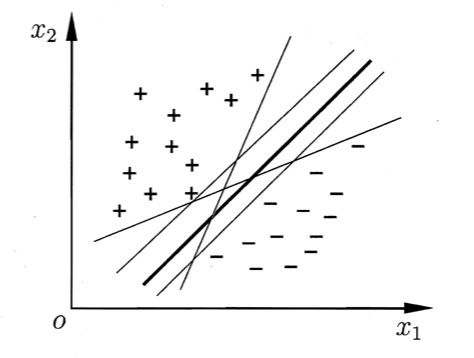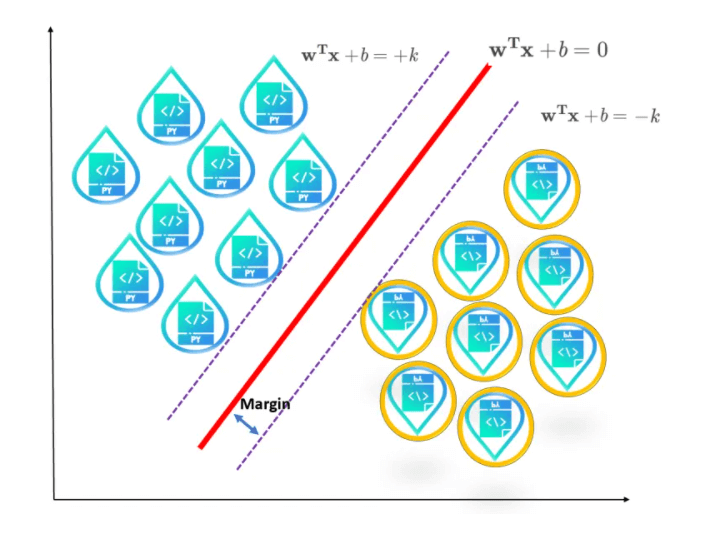# 【机器学习基础】第十六课：支持向量机之间隔与支持向量

## 二维平面，超平面，间隔，支持向量，支持向量机

Posted by x-jeff on September 14, 2020

【机器学习基础】系列博客为参考周志华老师的《机器学习》一书，自己所做的读书笔记。

# 1.理解超平面

## 1.1.二维平面

👉在三维x-y-z坐标系中，可以将平面定义为一个方程的集：

$ax+by+cz+d=0$

👉由一点和一个法向量决定的平面：

$ax+by+cz=ax_0+by_0+cz_0$

👉通过三点的平面：

$\begin{vmatrix} x-x_1 & y-y_1 & z-z_1 \\ x_2-x_1 & y_2-y_1 & z_2-z_1 \\ x_3-x_1 & y_3-y_1 & z_3-z_1 \\ \end{vmatrix}=0$

👉一点到平面的距离：

$D=\frac{\mid ax_1+by_1+cz_1+d \mid}{\sqrt {a^2+b^2+c^2}}$

👉判定两个平面平行：

$\alpha:A_1 x+B_1y+C_1z+D_1=0$ $\beta:A_2 x+B_2y+C_2z+D_2=0$

$\vec{n_1} // \vec{n_2}$

$\vec{n_1} = \lambda \vec{n_2}$

$A_1=\lambda A_2,B_1=\lambda B_2,C_1=\lambda C_2$

$\frac{A_1}{A_2}=\frac{B_1}{B_2}=\frac{C_1}{C_2}=\lambda$

👉两平行平面之间的距离：

$\alpha : ax+by+cz+d_1=0$ $\beta : ax+by+cz+d_2=0$

$\frac{\mid d_1 - d_2 \mid}{\sqrt{a^2+b^2+c^2}}$

## 1.2.超平面

$a_1x_1 + ...... + a_n x_n=b$

1.1部分中二维平面的性质也可推广到超平面。

# 2.间隔与支持向量$\mathbf w^T \mathbf x +b = 0$

$r=\frac{\mid \mathbf w^T \mathbf x +b \mid}{\lVert \mathbf w \rVert}$$\left\{ \begin{array}{c} \mathbf w^T \mathbf x_i +b \geqslant +k, y_i=+1 \\ \mathbf w^T \mathbf x_i +b \leqslant -k, y_i=-1 \\ \end{array} \right. \tag{1}$

$\gamma = \frac{2k}{\lVert \mathbf w \rVert}$

$\left\{ \begin{array}{c} \mathbf w^T \mathbf x_i +b \geqslant +1, y_i=+1 \\ \mathbf w^T \mathbf x_i +b \leqslant -1, y_i=-1 \\ \end{array} \right. \tag{2}$

$\gamma = \frac{2}{\lVert \mathbf w \rVert}$

\begin{align*} &\max \limits_{\mathbf w,b} \quad \frac{2}{\lVert \mathbf w \rVert} \\ & \begin{array}{r@{\quad}r@{}l@{\quad}l} s.t.& y_i(\mathbf w^T \mathbf x_i +b) \geqslant 1,i=1,2,...,m \\ \end{array} \end{align*}

\begin{align*} &\min \limits_{\mathbf w,b} \quad \frac{1}{2} \lVert \mathbf w \rVert ^2 \\ & \begin{array}{r@{\quad}r@{}l@{\quad}l} s.t.& y_i(\mathbf w^T \mathbf x_i +b) \geqslant 1,i=1,2,...,m \\ \end{array} \end{align*}

# 3.参考资料